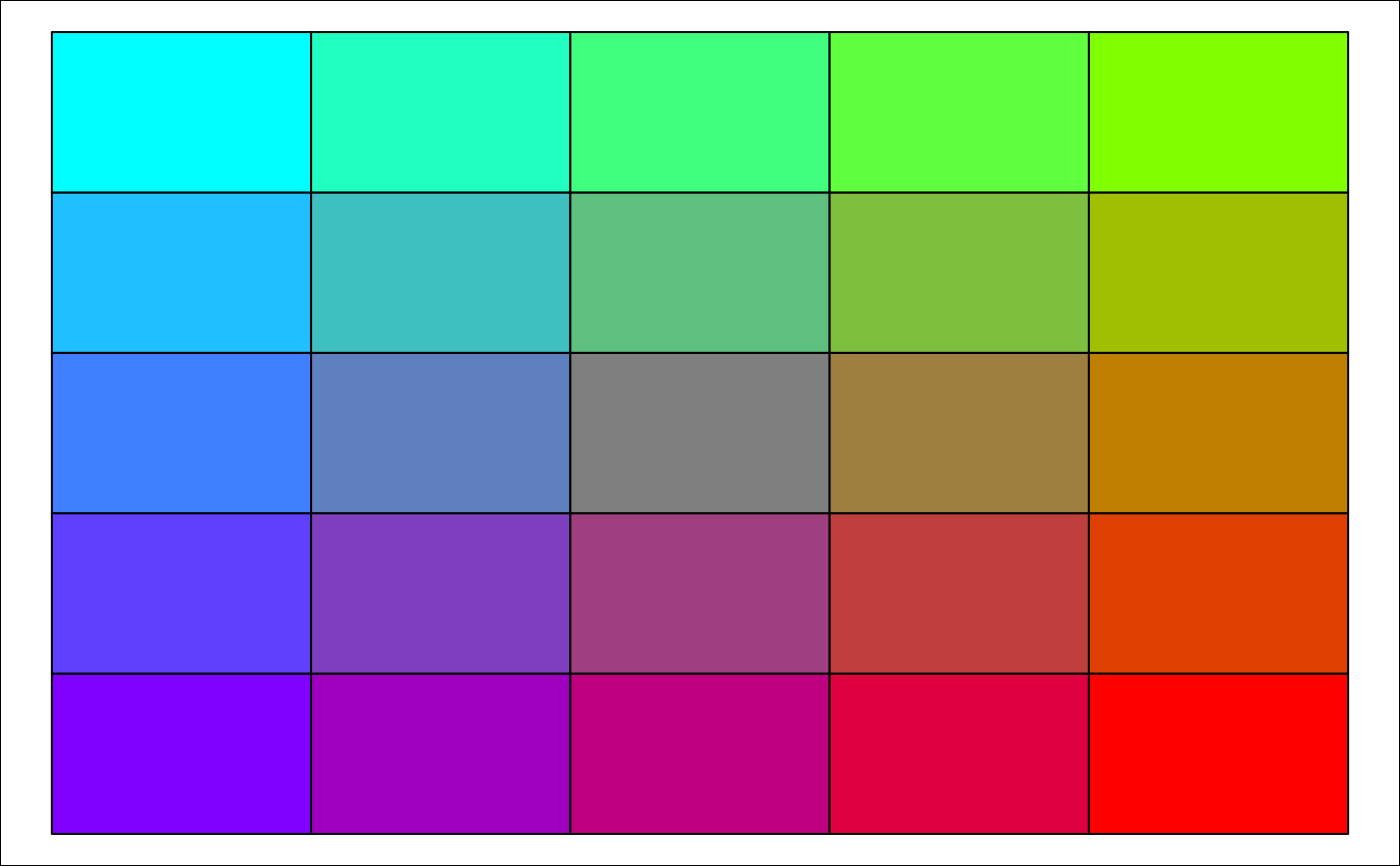Generates a 2D matrix of graduated colours by interpolating between the given colours specifying the four corners.

## Usage

``colour_mat(cols, n = c(10, 10), rotate, plot = FALSE)``

## Arguments

cols

vector of length >= 4 of colors (example, default = ```rainbow (4)```, or `RColorBrewer::brewer.pal (4, 'Set1')`). `cols` are wrapped clockwise around the corners from top left to bottom left.

n

number of rows and columns of colour matrix (default = 10; if length 2, then dimensions of rectangle).

rotate

rotates the entire colour matrix by the specified angle (in degrees).

plot

plots the colour matrix.

## Value

`Matrix` of colours.

`add_osm_groups`.

Other colours: `adjust_colours()`

## Examples

``````cm <- colour_mat (n = 5, cols = rainbow(4), rotate = 90, plot = TRUE)# 'colour_mat' is intended primarily for use in colouring groups added with
# 'add_osm_groups' using the 'colmat = TRUE' option:
bbox <- get_bbox (c (-0.13, 51.5, -0.11, 51.52))
# Generate random points to serve as group centres
set.seed (2)
ngroups <- 6
x <- bbox [1,1] + runif (ngroups) * diff (bbox [1,])
y <- bbox [2,1] + runif (ngroups) * diff (bbox [2,])
groups <- cbind (x, y)
groups <- apply (groups, 1, function (i)
sp::SpatialPoints (matrix (i, nrow = 1, ncol = 2)))
# plot a basemap and add groups
map <- osm_basemap (bbox = bbox, bg = "gray20")
map <- add_osm_groups (map, obj = london\$dat_BNR, group = groups,
cols = rainbow (4), colmat = TRUE, rotate = 90)
print_osm_map (map)
``````